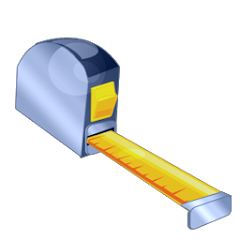# Imperial / Metric Converter +

1K+Everyone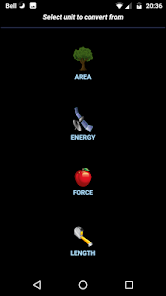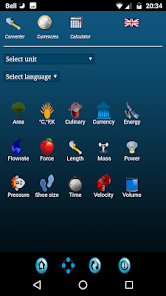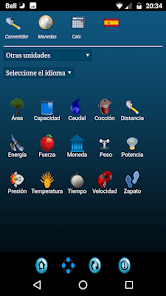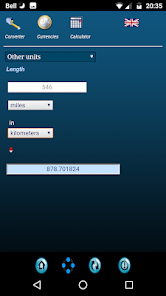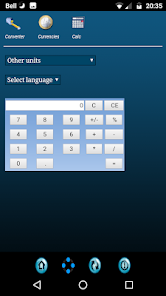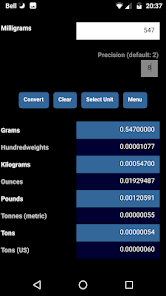Multilingual app for the conversion of currencies and units of measurement.

Supported languages: Albanian, Arabic, Chinese, Danish, Dutch, English, Finnish, French, German, Hebrew, Italian, Japanese, Korean, Norwegian, Portuguese, Russian, Spanish and Swedish.

The following types of measure units can be converted...

Area: acre, are, hectare (ha), square centimeter (cm2), square decimeter (dm2), square foot (ft2), square kilometer (km2), square meter (m2), square mile (mi2), square millimeter (mm2) and square yard (yd2).

Energy: British Thermal Unit (BTU), calorie (IST), calorie (thermo), cubic centimeter-atmosphere (cc-atm), foot-pound-force (lbf), gram-calorie, inch-pound-force, Joule, kilocalorie (kcal), kilogram-calorie, kilowatt per hour (kWh), pound-CHU, US horsepower per hour and watt per hour.

Flowrate: barrel per day, cubic centimeter per second (cc/s), cubic foot per minute, cubic foot per second, cubic inch per minute, cubic inch per second, cubic meter per hour, cubic yard per minute, gallon per day, gallon per minute, gallon per second, liter per minute, liter per second and miner's inch.

Force: crinal, dyne, grain, imperial ton (long ton), kilogram-force (kgf), kilogram-meter per square second, kip, Newton, ounce-force, poundal, pound-force and slug.

Length: centimeter (cm), chain, decimeter (dm), foot (ft), furlong, hectometer (hm), inch (in), kilometer (km), league units, meter (m), mile (mi), millimeter (mm), rod and yard (yd).

Mass: dram, grain, gram (g), hundredweight (cwt), kilogram (kg), milligram (mg), ounce, pound (lb), quarter, stone, ton, tonne and US ton.

Power: BTU per hour, BTU per minute, BTU per second, erg per second, foot-pound-force per hour, foot-pound-force per minute, foot-pound-force per second, gigawatt (GW), Joule per second, kilowatt (kW), megawatt (MW), metric horsepower, ton (refrigeration) and watt.

Pressure: bar, barye, inch H2O (15.56°C), inch Hg (15.56°C), kilogram-force per square centimeter, kilogram-force per square meter, kiloPascal (kPa), megaPascal (mPa), Pascal (Pa), pound per square foot, pound per square inch, poundal per square foot, poundal per square inch, short ton per square foot and torr.

Shoe size.

Temperature: Celsius, Fahrenheit and Kelvin.

Time: microsecond, millisecond, second, minute, hour, day, week, year, leap year, decade, century and millennium.

Velocity: centimeter per second (cm/s), foot per hour, foot per minute, foot per second, kilometer per hour (kmh), knot (kn), meter per minute, meter per second, mile per hour (mph) and mile per minute.

Volume: cubic centimeter (cc), cubic decimeter (dm3), cubic foot (ft3), cubic inch (in3), cubic meter (m3), cubic yard (yd3), fluid drachm, fluid ounce (fl oz), gallon, gill, liter (l), milliliter (ml), pint, quart, US gallon and US pint.

Ancient Greek and Roman units.

____________________________

Updated on
May 5, 2021

## Data safety

Safety starts with understanding how developers collect and share your data. Data privacy and security practices may vary based on your use, region, and age. The developer provided this information and may update it over time.No data shared with third parties